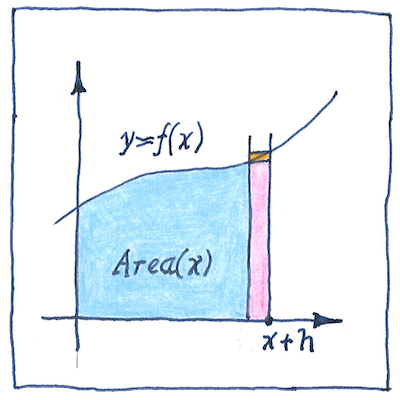# 1668

## The book of science

Tom Sharp

 James Gregory, Isaac Barrow mathematics

## Fundamental theorem of calculus

• Early Greek and Chinese mathematicians
• used the “method of exhaustion,”
• determining a value by disproving a series of approximations.
• This method foreshadowed the concept of a limit.
• A limit is the value
• that the result of a function approaches
• as its input approaches a given value.
• Determining limits gave European mathematicians
• the ability to prove the continuity of functions,
• and to determine their differentials and integrals.
• James Gregory and Isaac Barrow showed
• that the derivative and the integral
• are inverses of each other.
• The derivative of velocity is acceleration.
• The integral of acceleration is the velocity.

## Derivative and integral

• The derivative of a continuous function
• is the slope of the tangent at a point
• which is the same as the rate of change.
• For a function giving velocity over time,
• the derivative is acceleration at an instant.
• You can derive the derivative
• by considering the slope of a line between
• a target point and a nearby point on the curve
• and finding its slope when the distance
• between the points approaches zero.
• The integral of a continuous function
• is the area under the curve.
• For a function giving the velocity over time,
• because distance is velocity times time,
• the integral is the distance covered.
• You can derive the integral
• by considering adjacent rectangular slices
• whose sum approximates the total area under the curve
• and seeing what you get when the widths
• of the rectangles approaches zero.

## Limits

• Nearly everything has a limit
• and it’s generally not infinity.
• There are infinite points between zero and one,
• but you can measure their span with a ruler.
• Here I try not to be pessimistic,
• but there’s a limit to optimism.
• There’s a limit to how much I can afford.
• There’s a limit to how much I can stand.
• You live only once. Knowledge is power,
• but there’s a limit to it. Even limits have limits.
• A man’s reach should exceed his grasp,
• but there ought to be a limit to stupidity.

The work of many mathematicians led to the work of James Gregory and Isaac Barrow. A fuller history could name Eudoxus, Liu Hui, Archimedes, Zu Chongzhi, Alhazen, Pierre de Fermat, Bonaventura Cavalieri, Evangelista Torricelli, and John Wallis. Then there were Isaac Newton and Gottfried Wilhelm Leibniz, who invented calculus as a mathematics of motion and change.

See also in The book of science:

Readings on wikipedia: